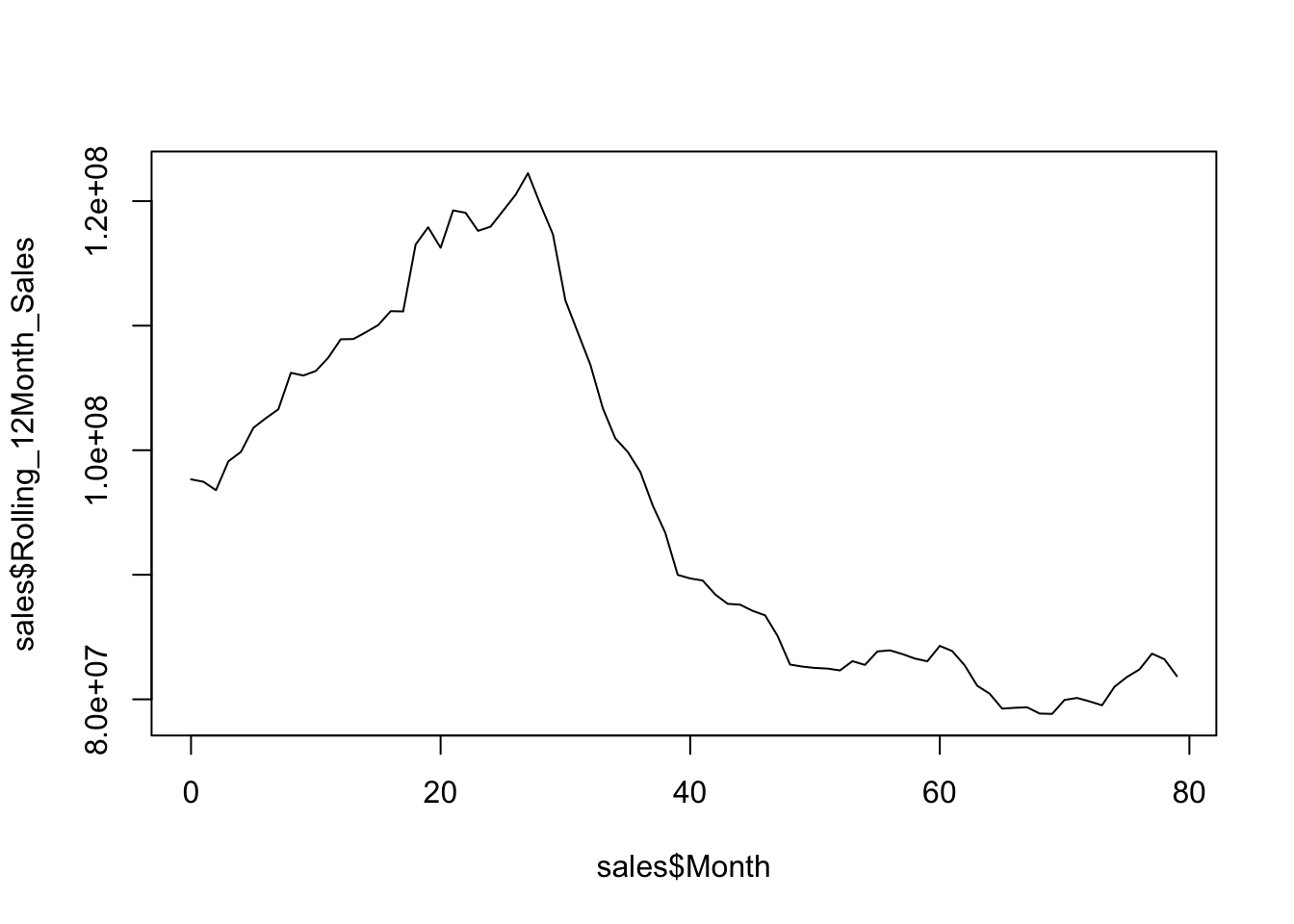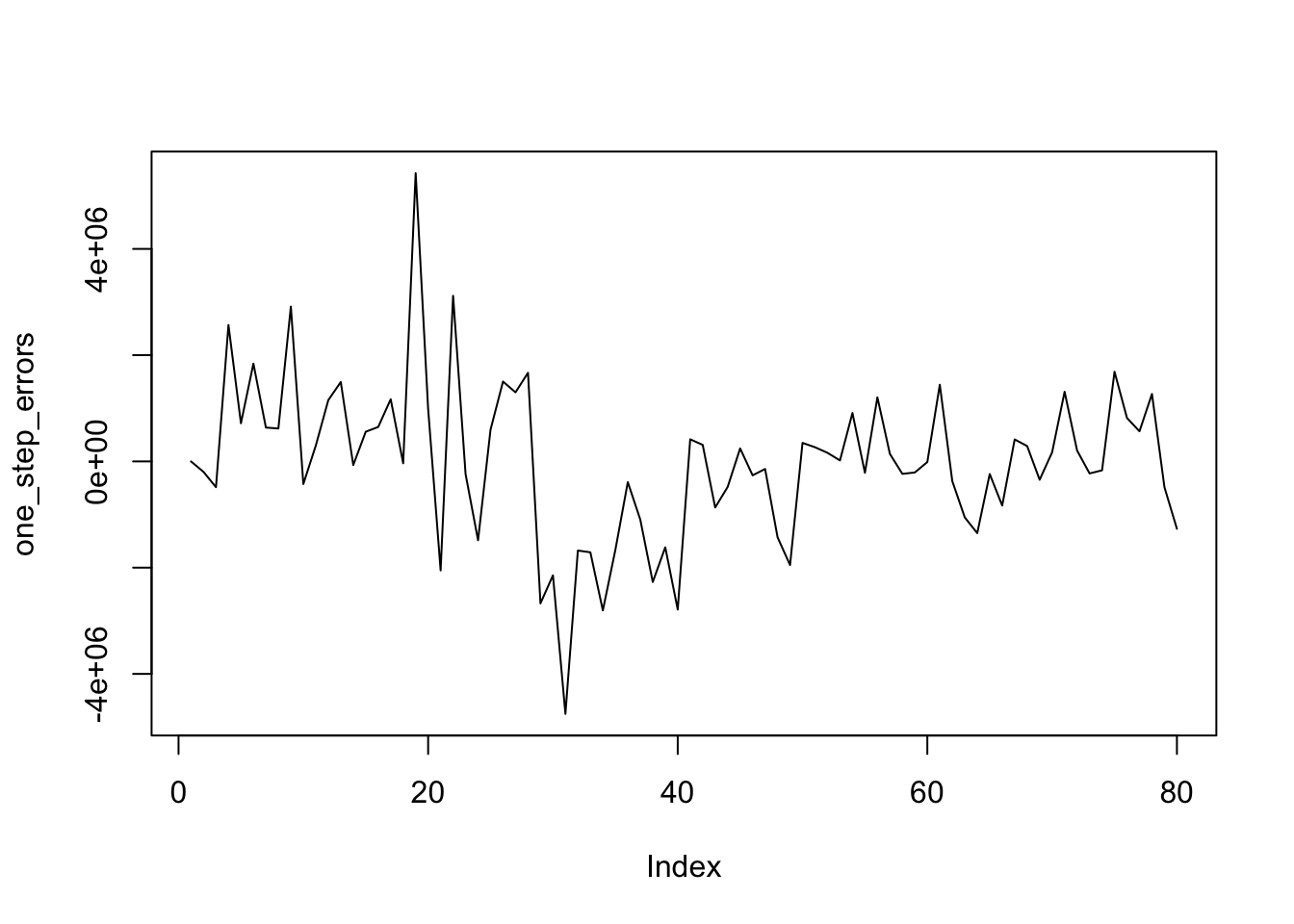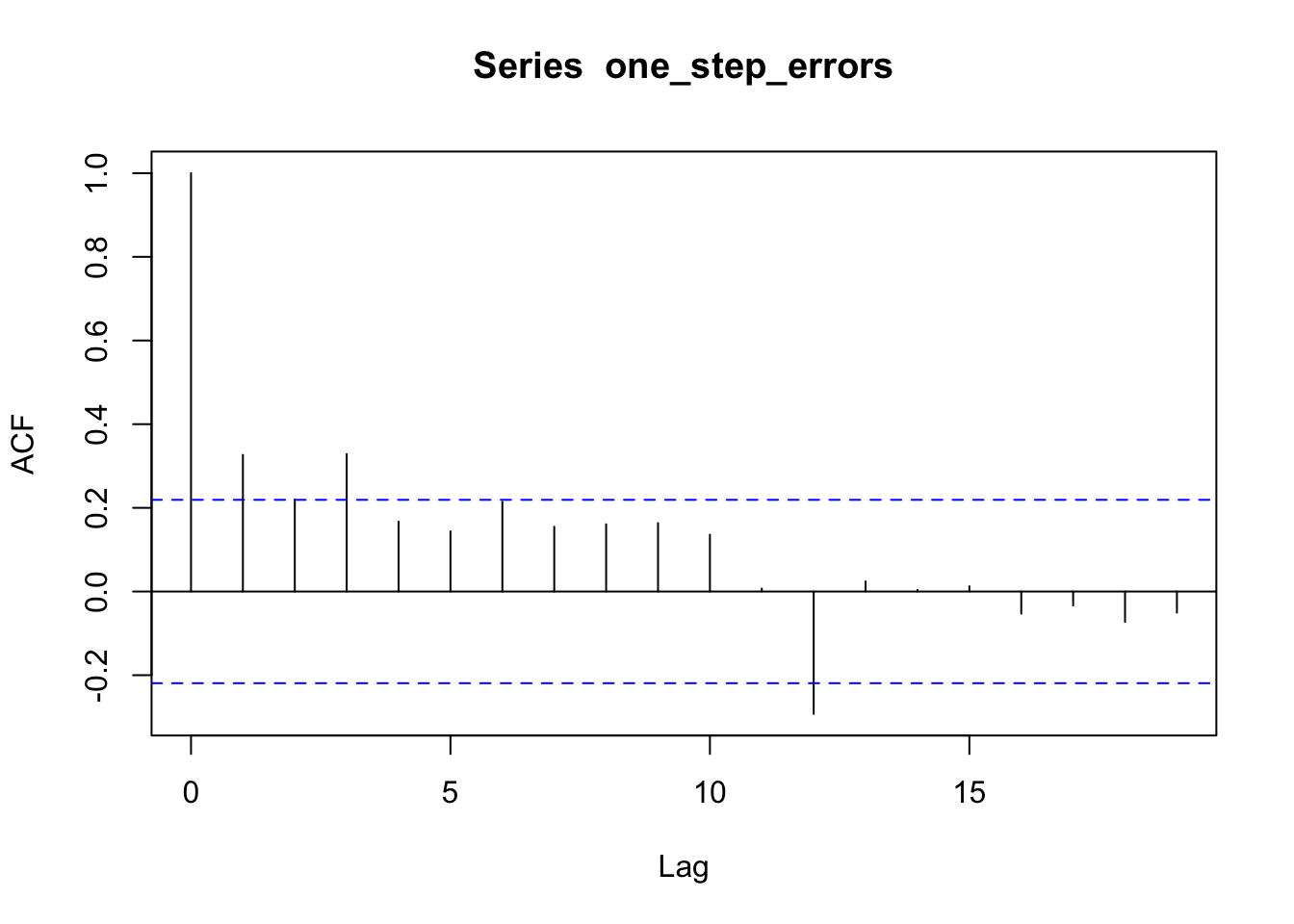## Purpose

This markdown provides the complete R code for the proposed solution of Sisifo’s page team to the MineThatData Forecasting Challenge. The challenge is to forecast the next 3 months of a target time series that contains data of sales of an unknown company for 80 months. The proposed solution uses Bayesian Structural Time Series models, through the excellent open source library `bsts` by Steve Scott.

This solution was published at this MineThatData blog post in Nov 15th 2017, and it got the best `squared error` of all solutions presented!!! (see here).

If you are interested on the theoretical justification of the solution, some background about BSTS models and the way to use them (in particular, setting up Bayesian priors and doing cross-validation for time series, i.e. forward chaining), you can check the whitepaper in pdf format that went along with the proposed solution.

The data can be read directly from the excel file using the code below. Note that in the original file a few cells are duplicated (the ones corresponding to the month 41) and these duplicates are removed. A simple plot of the data is also provided.

``````library(readxl)
library(httr)
GET("http://minethatdata.com/MineThatData_ForecastChallenge_20170914.xlsx",
write_disk(tf <- tempfile(fileext = ".xlsx")))``````
``````## Response [http://minethatdata.com/MineThatData_ForecastChallenge_20170914.xlsx]
##   Date: 2017-12-03 17:03
##   Status: 200
##   Size: 21.8 kB
## <ON DISK>  /var/folders/lm/6kvyzp5d3ds_20dxw939n5hw0000gp/T//RtmpTHH0nN/file4fb92f432430.xlsx``````
``````sales <-
col_names=TRUE, col_types=rep("numeric", 17))
sales <- sales[! duplicated(sales\$Month),]
plot(sales\$Month, sales\$Rolling_12Month_Sales, type="l")``````### Validation loop

How to setup the Bayesian priors for the model when you do not have clear priors? In the case of the Forecasting Challenge, there is not even any information at all about the business context. A possible strategies is to use `cross-validation`, but in a flavor adapted to time series, usually called `forward chaining` (see this cross-validated answer or this blog post).

The following code adopts the strategy for a `bsts` model, where:

• `data` is the full dataset
• `horizon` refers to the prediction horizon
• `number_of_folds` i.e. number of loops of `forward chaining`
• `ss.function` is a function for defining the `state.specification` of the `bsts` model; a function is needed since the `state.specification` component needs to be trained (it extracts some parameters from the available data, while the training data changes in every iteration of the loop)
• `niter`, `seed` and `burn`: see ?bsts
• the rest are for debugging and visualization

The loop implements 3 measures, the root mean square deviation, and some other measures commonly used in time series prediction, mape and mase.

``library(bsts)``
``````# cross-validation loop
bsts.cv.loop <- function(data,
horizon,
number_of_folds,
ss.function,
niter=1000,
seed=1232,
burn=250,
do.plot=TRUE,
verbose=TRUE,
debug=TRUE) {
rmse_v <- c()
mape_v <- c()
mase_v <- c()
for (fold in 1:number_of_folds) {
# construct data_train/data_test
l <- length(data) - fold*horizon
if (debug) print(l)
data_train <- data[1:l]
data_test <- data[(l+1):(l+horizon)]
# fit model & predict
model <- bsts(data_train,
state.specification=ss.function(data_train),
niter=1000,
seed=1232,
ping=0)
pred <- predict(model, horizon=horizon, burn=burn)
if (do.plot) {
# plot
plot(pred, plot.original = 36)
lines((l+1):(l+horizon), data_test, col="red", type="l")
}
# evaluation
errors <- data_test-pred\$mean
rmse <- sqrt(mean(errors^2))
rmse_v <- c(rmse_v, rmse)
mape <- mean(abs(errors)/data_test)*100
mape_v <- c(mape_v, mape)
naive_prediction_errors <- diff(data, 1)
mase <- mean(abs(errors)) / mean(abs(naive_prediction_errors))
mase_v <- c(mase_v, mase)
if (verbose) print(paste0("fold ", fold, ": mape ", mape, " / mase ", mase))
}
return(data.frame(rmse=rmse_v,mape=mape_v, mase=mase_v))
}``````

### Initialization

``````# 0. setup
# 0.1. target series to predict, ordered by month
target <- sales\$Rolling_12Month_Sales[order(sales\$Month)]
plot(target, type="l")

# 0.2. general parameters for the prediction
horizon=3 # as in Hillstron's challenge
number_of_folds=8 # reserves around 70% of the data for training only``````

### Selecting the right trend component and its parameters

After implementing the cross-validation loop, selecting the best trend component, together with its parameters, is a matter of brute force.

In the following loop, the validation is done for the `local level` trend component, which is the simplest and has only a parameter to set.

``````# 1.1. fit "local level" and set best prior values
res_df1 <- c()
for (sigma_guess in c(0.01, 0.1, 0.5)) {
res <-
bsts.cv.loop(target,
horizon=horizon,
number_of_folds=number_of_folds,
ss.function=function(data_train) {
sdy <- sd(data_train)
sd.prior <- SdPrior(sigma.guess=sigma_guess*sdy,
upper.limit=sdy,
sample.size=16)
data_train,
sigma.prior=sd.prior))
},
debug=FALSE,
verbose=FALSE)
print(paste0("sigma_guess ", sigma_guess,
": mean(rmse) ", mean(res\$rmse),
" / mean(mape) ", mean(res\$mape),
" / mean(mase) ", mean(res\$mase)))
res_row <- data.frame(sigma_guess=sigma_guess,
mean_rmse=mean(res\$rmse),
mean_mape=mean(res\$mape),
mean_mase=mean(res\$mase))
res_df1 <- rbind(res_df1, res_row)
}
res_df1
write.csv(res_df1, file="cv_res_local_level.csv",
row.names=FALSE)``````

This is the result. Differences of the fit for the values of the parameters are quite minimal.

``res_df1``
``````##   sigma_guess mean_rmse mean_mape mean_mase
## 1        0.01   1113079  1.296845 0.9144091
## 2        0.10   1113191  1.296731 0.9143498
## 3        0.50   1121630  1.302850 0.9187451``````

In a second loop, the `local linear` trend component is tested. In this one, two parameters are set in a nested loop.

``````# 1.2. fit "local linear" and set best prior values
res_df2 <- c()
for (level_sigma_guess in c(0.01, 0.1, 0.5)) {
for (slope_sigma_guess in c(0.01, 0.1, 0.5)) {
res <-
bsts.cv.loop(target,
horizon=horizon,
number_of_folds=number_of_folds,
ss.function=function(data_train) {
sdy <- sd(data_train)
sigma.prior <- SdPrior(sigma.guess=level_sigma_guess*sdy,
upper.limit=sdy,
sample.size=16)
slope.sigma.prior <- SdPrior(sigma.guess=slope_sigma_guess*sdy,
upper.limit=sdy,
sample.size=16)
data_train,
level.sigma.prior=sigma.prior,
slope.sigma.prior=slope.sigma.prior))
},
debug=FALSE,
verbose=FALSE)
print(paste0("level_sigma_guess ", level_sigma_guess, " / slope_sigma_guess ", slope_sigma_guess,
": mean(rmse) ", mean(res\$rmse),
" / mean(mape) ", mean(res\$mape),
" / mean(mase) ", mean(res\$mase)))
res_row <- data.frame(level_sigma_guess=level_sigma_guess,
slope_sigma_guess=slope_sigma_guess,
mean_rmse=mean(res\$rmse),
mean_mape=mean(res\$mape),
mean_mase=mean(res\$mase))
res_df2 <- rbind(res_df2, res_row)
}
}
res_df2
write.csv(res_df2, file="cv_res_local_linear_level.csv",
row.names=FALSE)``````

Note that this component works consistently worse than the `local level` for the series in hand.

``res_df2``
``````##   level_sigma_guess slope_sigma_guess mean_rmse mean_mape mean_mase
## 1              0.01              0.01   1364297  1.481599 1.0443783
## 2              0.01              0.10   1703694  1.840721 1.3013977
## 3              0.01              0.50   1744992  1.929831 1.3665115
## 4              0.10              0.01   1211363  1.331118 0.9428945
## 5              0.10              0.10   1577616  1.690251 1.1950030
## 6              0.10              0.50   1737569  1.918044 1.3579408
## 7              0.50              0.01   1244229  1.361862 0.9660299
## 8              0.50              0.10   1230068  1.311644 0.9268897
## 9              0.50              0.50   1624604  1.758403 1.2439698``````

Finally, the `semi-local linear` is tried. This is the most complex trend component available in the library, and has 4 parameters to set.

``````res_df3 <- c()
for (level_sigma_guess in c(0.01, 0.1, 0.5)) {
for (slope_mean_guess in c(0.01, 0.1, 0.5)) {
for (slope_sigma_guess in c(0.01, 0.1, 0.5)) {
for (ar_sigma_guess in c(0.01, 0.1, 0.5, 1)) {
res <-
bsts.cv.loop(target,
horizon=horizon,
number_of_folds=number_of_folds,
ss.function=function(data_train) {
sdy <- sd(data_train)
sigma.prior <- SdPrior(sigma.guess=level_sigma_guess*sdy,
upper.limit=sdy,
sample.size=16)
slope.mean.prior <- NormalPrior(mu=0,
sigma=slope_mean_guess*sdy)
slope.ar1.prior <- Ar1CoefficientPrior(mu=0,
sigma=1*ar_sigma_guess)
slope.sigma.prior <- SdPrior(sigma.guess=slope_sigma_guess*sdy,
upper.limit=sdy,
sample.size=16)
data_train,
level.sigma.prior=sigma.prior,
slope.mean.prior=slope.mean.prior,
slope.ar1.prior=slope.ar1.prior,
slope.sigma.prior=slope.sigma.prior))
},
debug=FALSE,
verbose=FALSE)
print(paste0("level_sigma_guess ", level_sigma_guess,
" / slope_mean_guess ", slope_mean_guess,
" / slope_sigma_guess ", slope_sigma_guess,
" / ar_sigma_guess ", ar_sigma_guess,
": mean(rmse) ", mean(res\$rmse),
" / mean(mape) ", mean(res\$mape),
" / mean(mase) ", mean(res\$mase)))
res_row <- data.frame(level_sigma_guess=level_sigma_guess,
slope_mean_guess=slope_mean_guess,
slope_sigma_guess=slope_sigma_guess,
ar_sigma_guess=ar_sigma_guess,
mean_rmse=mean(res\$rmse),
mean_mape=mean(res\$mape),
mean_mase=mean(res\$mase))
res_df3 <- rbind(res_df3, res_row)
}
}
}
}
res_df3
write.csv(res_df3, file="cv_res_semi_local_linear_level.csv",
row.names=FALSE)``````

The following are the values around the minimum. Note that there are only slightly better than the ones for the `local level` component; anyhow, the prediction with horizon 3 is going to be much more sensible for the `semi-local linear`, since the series is modeled as trend only.

``````res_df3[res_df3\$mean_mape <= min(res_df3\$mean_mape)*1.01 &
res_df3\$mean_mape >= min(res_df3\$mean_mape)*0.99,]``````
``````##    level_sigma_guess slope_mean_guess slope_sigma_guess ar_sigma_guess
## 14              0.01             0.10              0.01            0.1
## 26              0.01             0.50              0.01            0.1
## 39              0.10             0.01              0.01            0.5
## 40              0.10             0.01              0.01            1.0
## 51              0.10             0.10              0.01            0.5
## 63              0.10             0.50              0.01            0.5
## 64              0.10             0.50              0.01            1.0
##    mean_rmse mean_mape mean_mase
## 14   1102451  1.204493 0.8541107
## 26   1105388  1.205426 0.8548579
## 39   1111522  1.204680 0.8514994
## 40   1110975  1.204386 0.8512959
## 51   1102954  1.211028 0.8554464
## 63   1104017  1.211963 0.8562478
## 64   1102445  1.210628 0.8552218``````

### Chosen model

This is a final fit of the chosen model, with the trend component and its parameters as selected in the section above, for obtaining the predictions.

``````sdy <- sd(target)
level.sigma.prior <- SdPrior(sigma.guess=0.01*sdy,
upper.limit=sdy,
sample.size=16)
slope.ar1.prior <- Ar1CoefficientPrior(mu=0,
sigma=0.1)
slope.mean.prior <- NormalPrior(mu=0,
sigma=0.1*sdy)
slope.sigma.prior <- SdPrior(sigma.guess=0.01*sdy,
upper.limit=sdy,
sample.size=16)
target,
level.sigma.prior=level.sigma.prior,
slope.ar1.prior=slope.ar1.prior,
slope.mean.prior=slope.mean.prior,
slope.sigma.prior=slope.sigma.prior)
chosen_model <- bsts(target,
state.specification=state.specification,
niter=1000,
seed=1232)
pred <- predict(chosen_model, horizon = 3, burn = 250)``````

This is a simple analysis of the residuals; results look reasonable. There’s a very weak annual seasonality, already detected in the exploratory analysis, which is not captured by the trend-only model.

``````one_step_errors <- colMeans(chosen_model\$one.step.prediction.errors)
plot(one_step_errors, type="l")````````acf(one_step_errors)``And finally this is a plot of the predictions; certainty margins are quite wide, but still it looks reasonable for a trend-only model.

``plot(pred, plot.original = 80)``